How to calculate Square Roots and Cubic Roots

The functions sqrt and sometimes even cbrt are commonly available, but it is nice to see how they can be calculated.

There are several approaches, but the most popular ones are Newton’s method and an algorithmic formulation of how roots are taken manually, for those old enough to still have learned it in school. Earlier measurements that I did many years ago showed that the Newton approximation is slower, but it would be worth to do newer measurements.

So we have an equationor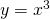and want to find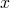or a well defined approximation ofwhen we know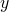. Mathematically speaking we want to assume thatis constant and we want to find anfor which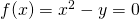or. If we guess such anand then draw the tangent at the curve of the function at the pointor, then the intersection point of the tangent can be used as the next approximation. This method converges in the case of these two functions (and some others) and is reasonably fast. Now the tangent has the linear equationwhere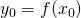and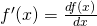is the derivative of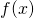. We want to solve this equation for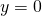and thus we get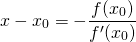and thus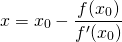As an iteration rule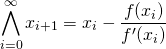In case of the sqare root we can just start with an estimation by shifting half the length to the right, but avoiding zero, which is important because of the division. Then we get for an appropriate n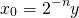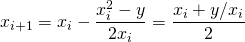The last form is quite intuitive, even without calculus. As I said this converges usefully fast and there is tons of math around to describe the behavior, speed, precision and convergence of the calculations performed in this algorithm. Written in Ruby just for integers, this is quite simple. Convergence is simply discovered by the fact that the result does not change any more, which may fail in some cases, where intermediate results oscillate between two values, but just for the purpose of benchmarking it seems to be sufficient:

def sqrt_newton(x)
if (x == 0) then
return 0
end
y0 = x
u0 = x
while (u0 > 0) do
y0 >>= 1
u0 >>= 2
end
y0 = [1, y0].max
yi = y0
yi_minus_1 = -1
loop do
yi_plus_1 = (yi + x/yi) >> 1;
if (yi_minus_1 == yi_plus_1) then
return [yi, yi_minus_1].min
elsif (yi == yi_plus_1) then
return yi
end
yi_minus_1 = yi
yi = yi_plus_1
end
end

The newton algorithm tends to oscillate between two approximations, so this termination criteria takes into account,and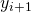and uses the lower of the two oscillating values. This results in calculating the largest integersuch that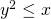and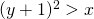.

For the third root we get for an appropriate n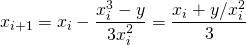Again this is a useful way and there is math around on when to stop for a desired precision.
Read Wikipedia for the convergence issues.

There is another approach, that people used to know when doing calculations on paper was more important than today. For the decimal system it works like this:

1. 7 3 2 0 5
----------------------
/ 3.00 00 00 00 00
/\/ 1 = 20*0*1+1^2
-
2 00
1 89 = 20*1*7+7^2
----
11 00
10 29 = 20*17*3+3^2
-----
71 00
69 24 = 20*173*2+2^2
-----
1 76 00
0 = 20*1732*0+0^2
-------
1 76 00 00
1 73 20 25 = 20*17320*5+5^2
----------
2 79 75
(source Wikipedia)
We group the digits to the left and to the right of the decimal point in groups of two. The highest possible square of an integral number that is below or equal to the leftmost group (03 in the example above) is used for the first digit of the result (1 in the example above). This square is subtracted and the next group is appended (200 in the example). Assuming thatis the result already calculated and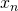is what we have achieved after the subtraction and the appending of the next group, we search for a digit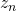such that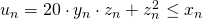.is chosen in such a way that it yields the maximum possiblewich is still. Subtractingfromand appending the next group allows for the next iteration.

Now this can be turned into an algorithm. The first approach is to just switch from decimal system to binary system. Then for each iteration step we have to deal just with the possible values of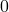and, which greatly simplifies the algorithm. Here is a simple ruby program that would do this:

def split_to_words(x, word_len)
bit_pattern = (1 << word_len) - 1   words = []   while (x != 0 || words.length == 0) do     w = x & bit_pattern     x = x >> word_len
words.unshift(w)
end
words
end

def sqrt_bin(x)
if (x == 0) then
return 0
end
xwords = split_to_words(x, 2)
xi = xwords - 1
yi = 1
1.upto(xwords.length-1) do |i|
xi = (xi << 2) + xwords[i]     d0 = (yi << 2) + 1     r  = xi - d0     b  = 0     if (r >= 0) then
b  = 1
xi = r
end
yi = (yi << 1) + b   end   return yi end

It seems that the two solutions yield the same results, but the sqrt_newton outperforms sqrt_bin by a factor of two.

Now we should reconsider, if base 2 is really the best choice. Actually we can use any power of 2 as a base and efficiently work with that. Apart from the initial first step, which is done by using an extended version of sqrt_bin, the next steps are estimated by division and trying neighboring values to get the exact result. This makes use of the fact that the equation we need to solve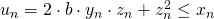with the maximumfullfilling this equation, where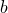is the base to which we are working, witch was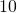or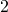above and could now be a power of. As soon as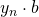has a certain size, the influence of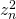becomes less relevant. We can consider the maximum posible value for z_n, which is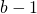and thus solve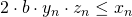and, each for the maximumfullfilling the equation. This can be calculated by simple division. If the range between the two solutions is small enough, then each value in the range can be tried to find the actual accurate solution for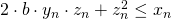and this is more efficient than working just bitwise. This method sqrt_word seems to outperform sqrt_newton for longer numbers, for example around 60 decimal digits with word_length=16. So the most promising approach seems to be to optimize the implementation and parameters of sqrt_word. The issue of termination, which has been properly addressed in the newton implementation, is already dealt with in this implementation. For more serious analysis it would be interesting to implement the algorithms in C or even in assembly language. So this is the final result for square roots, with some checks added:

def check_is_nonneg_int(x, name)
raise TypeError, "#{name}=#{x.inspect} must be Integer" unless (x.kind_of? Integer) && x >= 0
end

def check_word_len(word_len, name="word_len")
unless ((word_len.kind_of? Integer) && word_len > 0 && word_len <= 1024)
raise TypeError, "#{name} must be a positive number <= 1024"
end
end

def split_to_words(x, word_len)
check_is_nonneg_int(x, "x")
check_word_len(word_len)
bit_pattern = (1 << word_len) - 1
words = []
while (x != 0 || words.length == 0) do
w = x & bit_pattern
x = x >> word_len
words.unshift(w)
end
words
end

def sqrt_bin(x)
yy = sqrt_bin_with_remainder(x)
yy
end

def sqrt_bin_with_remainder(x)
check_is_nonneg_int(x, "x")
if (x == 0) then
return [0, 0]
end

xwords = split_to_words(x, 2)
xi = xwords - 1
yi = 1

1.upto(xwords.length-1) do |i|
xi = (xi << 2) + xwords[i]
d0 = (yi << 2) + 1
r  = xi - d0
b  = 0
if (r >= 0) then
b  = 1
xi = r
end
yi = (yi << 1) + b
end
return [yi, xi]
end

def sqrt_word(x, n = 16)
check_is_nonneg_int(x, "x")
check_is_nonneg_int(n, "n")

n2 = n << 1
n1 = n+1
check_word_len(n2, "2*n")
if (x == 0) then
return 0
end

xwords = split_to_words(x, n2)
if (xwords.length == 1) then
return sqrt_bin(xwords)
end

xi = (xwords << n2) + xwords
a  = sqrt_bin_with_remainder(xi)
yi = a
if (xwords.length <= 2) then
return yi
end

xi = a
2.upto(xwords.length-1) do |i|
xi = (xi << n2) + xwords[i]
d0 = (yi << n1)
q  = (xi / d0).to_i
j  = 10
was_negative = false
while (true) do
d = d0 + q
r = xi - (q * d)
break if (0 <= r && (r < d || was_negative))
if (r < 0) then
was_negative = true
q = q-1
else
q = q+1
end
j -= 1
if (j <= 0) then
break
end
end
xi = r
yi = (yi << n) + q
end
return yi
end

def sqrt_newton(x)
check_is_nonneg_int(x, "x")
if (x == 0) then
return 0
end
y0 = x
u0 = x
while (u0 > 0) do
y0 >>= 1
u0 >>= 2
end
y0 = [1, y0].max
yi = y0
yi_minus_1 = -1
loop do
yi_plus_1 = (yi + x/yi) >> 1;
if (yi_minus_1 == yi_plus_1) then
return [yi, yi_minus_1].min
elsif (yi == yi_plus_1) then
return yi
end
yi_minus_1 = yi
yi = yi_plus_1
end
end

This is the approach that has been built into the LongDecimal library, ignoring Newton. The examples have been added to github.

The algorithms can be extended to cubic roots or any higher roots. In this case, the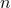th root ofis calculated by starting with the maximal integral number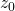with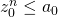and the subsequently finding numbers z_j fullfilling an equation of the form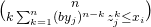. This is always easy to handle for base two, by just testing the two possible solutions. For higher bases and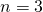it involves solving an quadratic equation, once the numbers are high enough to neglect the term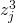. For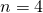it is just possible to take the square root of the square root. For higher values ofand bases other thanit becomes really difficult to tame this algorithm. So I intend to constrain myself to square roots and cube roots. I have not explored, if it is useful to calculate the cube root with a higher base thanand which approach provides the best performance for cube roots. Even the square root calculation can possibly be tuned a bit. Maybe this will be addressed in another article.